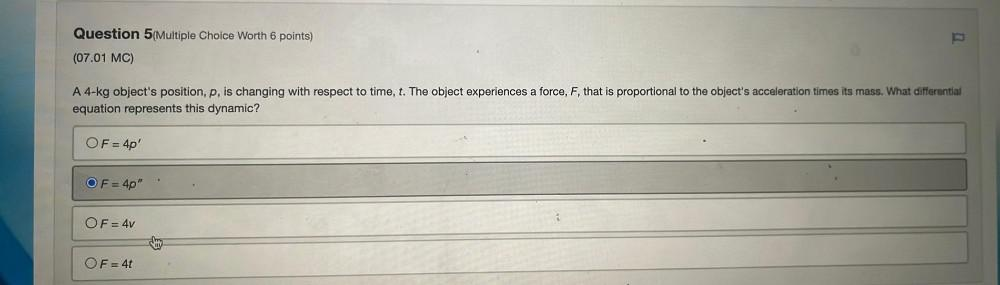Question:

# A 4-kg object's position, p, is changing with respect to

Last updated: 7/26/2022A 4-kg object's position, p, is changing with respect to time, t. The object experiences a force, F, that is proportional to the object's acceleration times its mass. What differential equation represents this dynamic? A. F = 4p' B. F = 4p" C. F = 4v D. F = 4t